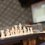# Select a number (Statistics)

This note is part of the set Select a number. Check out the rules here.

Total Points :

$1$ Yash Singhal $304.5$

$2$ Math Man $292.04$

$3$ Tan Li Xuan $290.17$

However, as there are significantly less people in the last round, the points obtained are quite high (outliers). So, the top $3$ for the first $9$ rounds are worth pointing out too.

$1$ Mursalin Habib $236.78$

$2$ Anuj Shikarkhane $234.26$

$3$ Yash Singhal $221.78$

Kudos for Yash Singhal for being in the top $3$ for both all rounds and Rounds $1 - 9$ despite only participating in a total of $8$ rounds!

Next, we have a look at the mean.

Technically, the $3$ highest mean scorers would be Sai Prasanth Pao, Ayush Vardhan Sinha and Pang Robin. (with a huge lead)

However, since they only participated in only one round, so the mean might not be accurate (especially only Round $10$)

So, we list only those who participated in at least $3$ rounds as a general law :

$1$ Krishna Sharma $49.23$

$2$ Utkarsh Dwivedi $44.9$

$3$ Yash Singhal $38.06$

Also, since the last round is quite an outlier, we'll list the top for the first $9$ rounds.

$1$ Dhruv Bhasin $31.7$

$2$ Yash Singhal $31.68$

$3$ Kinshuk Singh $31.155$

Also, we also list those who participated in all rounds :

Tan Li Xuan

Math Man

Thanks for all other participants for your support so that Select a number can run successfully. Thanks!Note by Zi Song Yeoh
6 years, 9 months ago

This discussion board is a place to discuss our Daily Challenges and the math and science related to those challenges. Explanations are more than just a solution — they should explain the steps and thinking strategies that you used to obtain the solution. Comments should further the discussion of math and science.

When posting on Brilliant:

• Use the emojis to react to an explanation, whether you're congratulating a job well done , or just really confused .
• Ask specific questions about the challenge or the steps in somebody's explanation. Well-posed questions can add a lot to the discussion, but posting "I don't understand!" doesn't help anyone.
• Try to contribute something new to the discussion, whether it is an extension, generalization or other idea related to the challenge.

MarkdownAppears as
*italics* or _italics_ italics
**bold** or __bold__ bold
- bulleted- list
• bulleted
• list
1. numbered2. list
1. numbered
2. list
Note: you must add a full line of space before and after lists for them to show up correctly
paragraph 1paragraph 2

paragraph 1

paragraph 2

[example link](https://brilliant.org)example link
> This is a quote
This is a quote
    # I indented these lines
# 4 spaces, and now they show
# up as a code block.

print "hello world"
# I indented these lines
# 4 spaces, and now they show
# up as a code block.

print "hello world"
MathAppears as
Remember to wrap math in $$ ... $$ or $ ... $ to ensure proper formatting.
2 \times 3 $2 \times 3$
2^{34} $2^{34}$
a_{i-1} $a_{i-1}$
\frac{2}{3} $\frac{2}{3}$
\sqrt{2} $\sqrt{2}$
\sum_{i=1}^3 $\sum_{i=1}^3$
\sin \theta $\sin \theta$
\boxed{123} $\boxed{123}$

Sort by:

Interesting Game ......Alas! I missed it

- 6 years, 9 months ago

I participated only in 3 :(

- 6 years, 9 months ago

But you have the highest mean overall! Congrats!

- 6 years, 9 months ago

I can't believe I forgot to submit in the last round!

- 6 years, 9 months ago

Ya, if you did, I think you'll definitely get #1 as the last round was a serious outlier. Still, you are still #1 most of the time and after 9 rounds. :)

- 6 years, 9 months ago